# area

Filled area 2-D plot

•## Syntax

``area(X,Y)``
``area(Y)``
``area(___,basevalue)``
``area(___,Name,Value)``
``area(ax,___)``
``a = area(___)``

## Description

example

````area(X,Y)` plots the values in `Y` against the x-coordinates `X`. The function then fills the areas between the curves based on the shape of `Y`: If `Y` is a vector, the plot contains one curve. `area` fills the area between the curve and the horizontal axis.If `Y` is a matrix, the plot contains one curve for each column in `Y`. `area` fills the areas between the curves and stacks them, showing the relative contribution of each row element to the total height at each x-coordinate. ```

example

````area(Y)` plots `Y` against an implicit set of x-coordinates and fills the areas between the curves. If `Y` is a vector, the x-coordinates range from 1 to `length(Y)`.If `Y` is a matrix, the x-coordinates range from 1 to the number of rows in `Y`. ```

example

````area(___,basevalue)` specifies the baseline value for the area plot. `basevalue` corresponds to a horizontal baseline. `area` fills the area confined between the curves and this line. Specify `basevalue` as the last argument in any of the previous syntaxes.```

example

````area(___,Name,Value)` modifies the properties of the area plot using one or more name-value pair arguments. The properties apply to all of the displayed areas. For example, `'LineStyle','--'` specifies a dashed line style for the plot. Specify the name-value pairs after all of the arguments in any of the previous syntaxes. For a list of properties, see Area Properties.```

example

````area(ax,___)` displays the area plot in the target axes. Specify the axes as the first argument in any of the previous syntaxes.```

example

````a = area(___)` returns one or more `Area` objects. The number of objects is equal to the number of plotted areas. Use `a` to modify properties of the areas after creating them. For a list of properties, see Area Properties.```

## Examples

collapse all

Create a vector of four values. Display the values in an area plot.

```y = [1 5 6 3]; area(y)```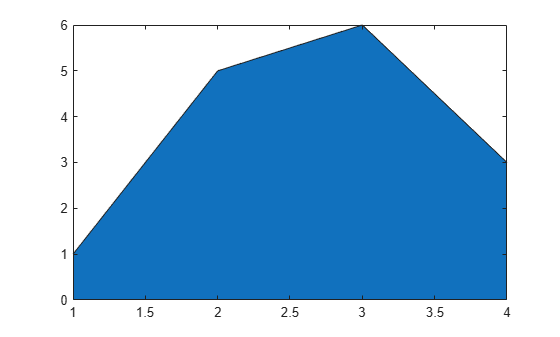Create matrix `Y`. Then display the values in `Y` as an area plot. Because `Y` contains three columns, `area` plots three curves and stacks them.

```Y = [1 5 3; 3 2 7; 1 5 3; 2 6 1]; area(Y)```Define `x` as a vector of three car dealership IDs. Define `Y` as a matrix containing the number of cars sold per model. Display the values in the matrix in an area plot. Then add the axis labels and a legend.

```x = [10 11 12]; Y = [21.6 25.4; 70.8 66.1; 58.0 43.6]; area(x,Y) xlabel('Dealership ID') ylabel('Sales') legend({'Model A','Model B'})```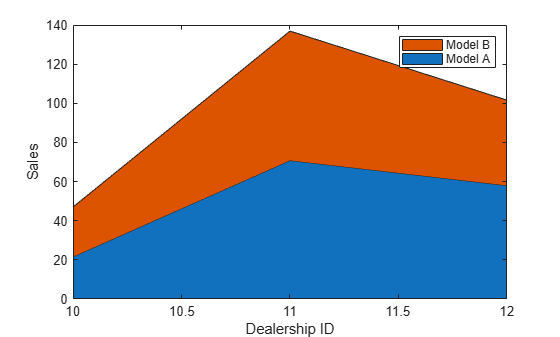Set the tick marks along the x-axis to correspond to the values in `x`.

```ax = gca; % current axes ax.XTick = x;```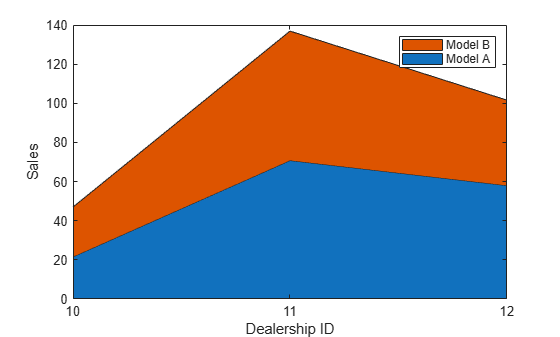Create matrix `Y`. Then display the values of `Y` in an area plot with a baseline value of `-4`. `area` fills the areas specified by the curves and the line `y = -4`.

```Y = [1 5 3; 3 2 7; 1 5 3; 2 6 1]; basevalue = -4; area(Y,basevalue)```Create matrix `Y`. Display the values of `Y` in an area plot that uses a dotted line style.

```Y = [1 3 5; 3 2 7; 3 4 2]; area(Y,'LineStyle',':')```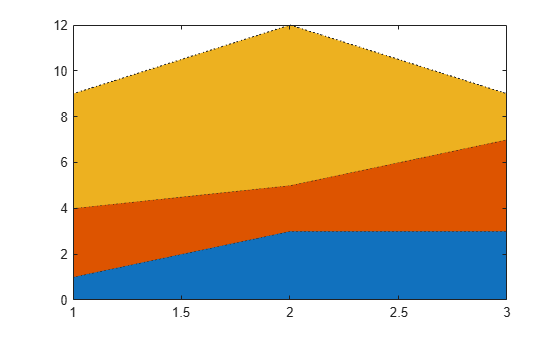Create a tiled chart layout in the `'flow'` tile arrangement, so that the axes fill the available space in the layout. Next, call the `nexttile` function to create an `Axes` object and return it as `ax1`. Display an area plot by passing `ax1` to the `area` function.

```tiledlayout('flow') ax1 = nexttile; Y1 = [3 6; 1 5; 7 2; 5 9]; area(ax1,Y1)```Repeat the process to create a second `Axes` object and a second area plot.

```ax2 = nexttile; Y2 = [4 2 11; 5 6 0; 1 7 2; 9 5 9]; area(ax2,Y2)```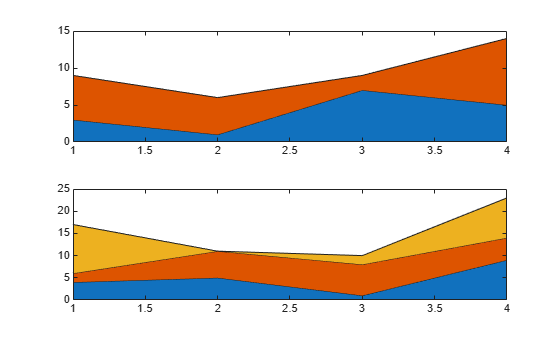Create matrix `Y`. Then create an area plot, specifying an output argument when calling the `area` function. In this case, `area` returns a vector of three `Area` objects. Each object corresponds to a different column of `Y`.

```Y = [2 3 4; 6 1 5; 7 4 9]; a = area(Y);```Modify the second area to be green with thick red edges.

```a(2).FaceColor = [0.2 0.6 0.5]; a(2).EdgeColor = [0.63 0.08 0.18]; a(2).LineWidth = 2;```Display an area plot with three curves.

`area([1 5 3; 3 2 7; 1 5 3; 2 6 1])`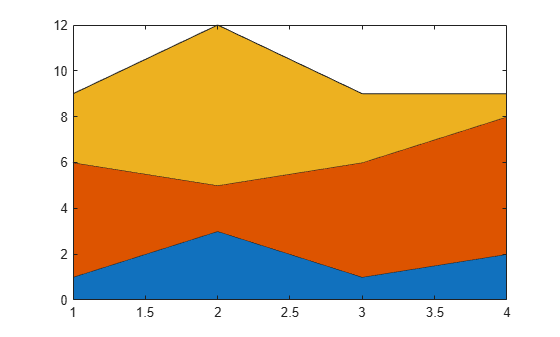Set the color order to blue, purple, and gray.

```newcolors = [0 0.5 1; 0.5 0 1; 0.7 0.7 0.7]; colororder(newcolors)```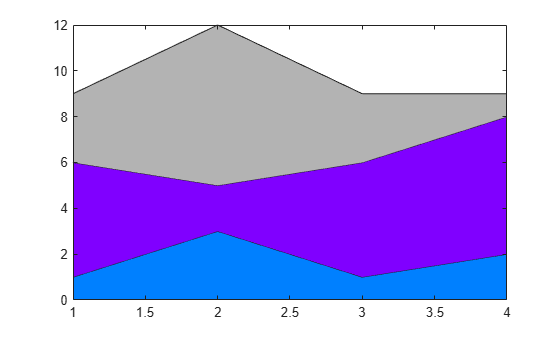## Input Arguments

collapse all

x-coordinates, specified as a vector or matrix. The size and shape of `X` depend on the shape of your data and the type of plot you want to create. This table describes the most common situations.

Type of PlotHow to Specify Coordinates
Single area

Specify `X` and `Y` as any combination of row or column vectors of the same length. For example:

`area([1 3 5],[9; 4; 6])`
Specify `X` as a vector of increasing values. If the values in `X` are not increasing, then `area` sorts the values before plotting.

Stacked areas

`area` plots one filled area for each column of `Y` and stacks the areas. Specify `Y` as a matrix and `X` as a row or column vector with length equal to the number of rows in `Y`. For example:

`area([1 2 3 4],[3 6; 1 5; 7 2; 5 9])`
If the values in `X` are not increasing, then `area` sorts the values before plotting.

You also can specify `X` as a matrix with the same size as `Y`. To avoid unexpected output when `X` is a matrix, specify `X` with identical columns.

Data Types: `single` | `double` | `int8` | `int16` | `int32` | `int64` | `uint8` | `uint16` | `uint32` | `uint64` | `categorical` | `datetime` | `duration`

y-coordinates, specified as a vector or matrix. The size and shape of `Y` depend on the shape of your data and the type of plot you want to create. This table describes the possible situations.

Type of PlotHow to Specify Coordinates
Single area

Specify `X` and `Y` as any combination of row or column vectors of the same length. For example:

`area([1 3 5],[9; 4; 6])`
Specify `X` as a vector of increasing values. If the values in `X` are not increasing, then `area` sorts the values before plotting.

Stacked areas

`area` plots one filled area for each column of `Y` and stacks the areas. Specify `Y` as a matrix and `X` as a row or column vector with length equal to the number of rows in `Y`. For example:

`area([1 2 3 4],[3 6; 1 5; 7 2; 5 9])`
If the values in `X` are not increasing, then `area` sorts the values before plotting.

You also can specify `X` as a matrix with the same size as `Y`. To avoid unexpected output when `X` is a matrix, specify `X` with identical columns.

Data Types: `single` | `double` | `int8` | `int16` | `int32` | `int64` | `uint8` | `uint16` | `uint32` | `uint64` | `duration`

Baseline value, specified as a numeric scalar. The baseline value specifies the y-coordinate of a horizontal baseline. `area` fills the area confined between the data curves and this baseline.

Target axes, specified as an `Axes` object. If you do not specify the axes, then `area` displays the plot in the current axes.

### Name-Value Arguments

Specify optional comma-separated pairs of `Name,Value` arguments. `Name` is the argument name and `Value` is the corresponding value. `Name` must appear inside quotes. You can specify several name and value pair arguments in any order as `Name1,Value1,...,NameN,ValueN`.

Example: `area([1 2 3],'FaceColor','r')` specifies a red fill color for the area.

Note

The properties listed here are only a subset. For a complete list, see Area Properties.

Area fill color, specified as an RGB triplet, a hexadecimal color code, a color name, or `'flat'`.

Starting in R2017b, the default value is an RGB triplet from the `ColorOrder` property of the axes. In previous releases, the default value was `'flat'` and the colors were based on the colormap.

For a custom color, specify an RGB triplet or a hexadecimal color code.

• An RGB triplet is a three-element row vector whose elements specify the intensities of the red, green, and blue components of the color. The intensities must be in the range `[0,1]`; for example, ```[0.4 0.6 0.7]```.

• A hexadecimal color code is a character vector or a string scalar that starts with a hash symbol (`#`) followed by three or six hexadecimal digits, which can range from `0` to `F`. The values are not case sensitive. Thus, the color codes `'#FF8800'`, `'#ff8800'`, `'#F80'`, and `'#f80'` are equivalent.

Alternatively, you can specify some common colors by name. This table lists the named color options, the equivalent RGB triplets, and hexadecimal color codes.

Color NameShort NameRGB TripletHexadecimal Color CodeAppearance
`'red'``'r'``[1 0 0]``'#FF0000'``'green'``'g'``[0 1 0]``'#00FF00'``'blue'``'b'``[0 0 1]``'#0000FF'``'cyan'` `'c'``[0 1 1]``'#00FFFF'``'magenta'``'m'``[1 0 1]``'#FF00FF'``'yellow'``'y'``[1 1 0]``'#FFFF00'``'black'``'k'``[0 0 0]``'#000000'``'white'``'w'``[1 1 1]``'#FFFFFF'``'none'`Not applicableNot applicableNot applicableNo color

Here are the RGB triplets and hexadecimal color codes for the default colors MATLAB® uses in many types of plots.

`[0 0.4470 0.7410]``'#0072BD'``[0.8500 0.3250 0.0980]``'#D95319'``[0.9290 0.6940 0.1250]``'#EDB120'``[0.4940 0.1840 0.5560]``'#7E2F8E'``[0.4660 0.6740 0.1880]``'#77AC30'``[0.3010 0.7450 0.9330]``'#4DBEEE'``[0.6350 0.0780 0.1840]``'#A2142F'`Area outline color, specified as an RGB triplet, a hexadecimal color code, a color name, or `'flat'`. Specifying this property as `'flat'` uses the colors of the colormap.

For a custom color, specify an RGB triplet or a hexadecimal color code.

• An RGB triplet is a three-element row vector whose elements specify the intensities of the red, green, and blue components of the color. The intensities must be in the range `[0,1]`; for example, ```[0.4 0.6 0.7]```.

• A hexadecimal color code is a character vector or a string scalar that starts with a hash symbol (`#`) followed by three or six hexadecimal digits, which can range from `0` to `F`. The values are not case sensitive. Thus, the color codes `'#FF8800'`, `'#ff8800'`, `'#F80'`, and `'#f80'` are equivalent.

Alternatively, you can specify some common colors by name. This table lists the named color options, the equivalent RGB triplets, and hexadecimal color codes.

Color NameShort NameRGB TripletHexadecimal Color CodeAppearance
`'red'``'r'``[1 0 0]``'#FF0000'``'green'``'g'``[0 1 0]``'#00FF00'``'blue'``'b'``[0 0 1]``'#0000FF'``'cyan'` `'c'``[0 1 1]``'#00FFFF'``'magenta'``'m'``[1 0 1]``'#FF00FF'``'yellow'``'y'``[1 1 0]``'#FFFF00'``'black'``'k'``[0 0 0]``'#000000'``'white'``'w'``[1 1 1]``'#FFFFFF'``'none'`Not applicableNot applicableNot applicableNo color

Here are the RGB triplets and hexadecimal color codes for the default colors MATLAB uses in many types of plots.

`[0 0.4470 0.7410]``'#0072BD'``[0.8500 0.3250 0.0980]``'#D95319'``[0.9290 0.6940 0.1250]``'#EDB120'``[0.4940 0.1840 0.5560]``'#7E2F8E'``[0.4660 0.6740 0.1880]``'#77AC30'``[0.3010 0.7450 0.9330]``'#4DBEEE'``[0.6350 0.0780 0.1840]``'#A2142F'`Line style, specified as one of the options listed in this table.

Line StyleDescriptionResulting Line
`'-'`Solid line`'--'`Dashed line`':'`Dotted line`'-.'`Dash-dotted line`'none'`No lineNo line

Area outline width, specified as a scalar numeric value in point units. One point equals 1/72 inch.

Example: `1.5`

Data Types: `single` | `double` | `int8` | `int16` | `int32` | `int64` | `uint8` | `uint16` | `uint32` | `uint64`

## Tips

• `area` uses colors based on the `ColorOrder` property of the axes. It cycles through all colors, and then it repeats the cycle if you plot more filled regions than there are colors.

Starting in R2019b, you can change the colors after plotting by setting the `ColorOrder` property on the axes. You can also call the `colororder` function to change the color order for all the axes in the figure.

## Extended Capabilities

### Topics

Introduced before R2006a